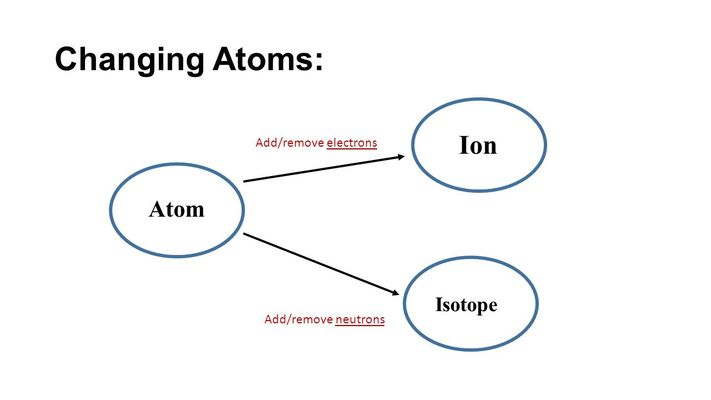Ions and Isotopes
4 years ago
mrlongvb
Save
Edit
Host a game
Live GameLive
Homework
Solo Practice
Practice17 QuestionsShow answers
• Question 1
30 seconds
Q. Negative ions are called
Cations
Electrons
Anions
Neutrons
• Question 2
30 seconds
Q. When an atom loses a valence electron, it becomes a(n) _____________ ion.
positive
negative
neutral
polyatomic
• Question 3
30 seconds
Q. Positive ions form when atoms _________ valence electrons.
lose
gain
share
gain or share
• Question 4
30 secondsQ. How many protons are in this element?
79
196
117
Cannot be determined
• Question 5
30 seconds
Q. What charge does an atom have if it LOSES an electron?
Positive (+)
Negative (-)
• Question 6
30 seconds
Q. When an atom LOSES an electron it is a
cation
anion
• Question 7
60 seconds
Q. Ions are:
atoms with a positive or negative charge
atoms with no charge
atoms with ONLY a positive charge
atoms with ONLY a negative charge
• Question 8
30 seconds
Q. A negative ion is a
cation
anion
• Question 9
60 seconds
Q. Valence electrons are:
Electrons farthest away from the nucleus
Electrons closest to the nucleus
Electrons that just come and go - they don't stay with the atom
• Question 10
30 seconds
Q. What charge does an atom have if it GAINS an electrons?
Positive (+)
Negative (-)
• Question 11
30 secondsQ. What is the number of protons?
34
16
18
S
• Question 12
30 secondsQ. What is the number of neutrons?
34
79
45
35
• Question 13
30 seconds
Q. If you have 10 protons and 9 neutrons, what would the mass number be?
19
9
10
1
• Question 14
30 seconds
Q. What is the definition of isotope?
atoms of an element with different numbers of neutrons.
materials break down over time at a very consistent rate
• Question 15
30 secondsQ. What do these isotopes of carbon all have in common?
neutrons & mass number
atomic number and neutrons
atomic number and electrons
protons, atomic number, and mass number
• Question 16
30 seconds
Q. What are the names of the horizontal rows on the periodic table?
The periods
The Groups
The Non-Metals
Ionization Energies
• Question 17
30 seconds
Q. What is an example of an Isotope